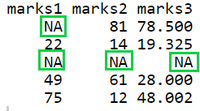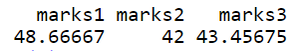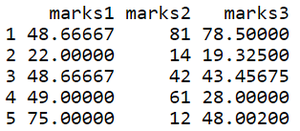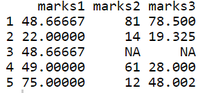Open in App
Not now

# Replace Missing Values by Column Mean in R DataFrame

• Last Updated : 17 Oct, 2021

In this article, we are going to see how to replace missing values with columns mean in R Programming Language. Missing values in a dataset are usually represented as NaN or NA. Such values must be replaced with another value or removed. This process of replacing another value in place of missing data is known as Data Imputation

## R

 `# creating a dataframe``data <- ``data.frame``(marks1 = ``c``(``NA``, 22, ``NA``, 49, 75),``                   ``marks2 = ``c``(81, 14, ``NA``, 61, 12),``                   ``marks3 = ``c``(78.5, 19.325, ``NA``, 28, 48.002))``data`

Output:## Method 1: Replace columns using mean() function

Let’s see how to impute missing values with each column’s mean using a dataframe and mean( ) function. mean() function is used to calculate the arithmetic mean of the elements of the numeric vector passed to it as an argument.

Syntax of mean() : mean(x, trim = 0, na.rm = FALSE, …)

Arguments:

• x – any object
• trim – observations to be trimmed from each end of x before the mean is computed
• na.rm – FALSE to remove NA values

## R

 `# compute each column's mean using mean() function``m <- ``c``()``for``(i ``in` `colnames``(data)){``  ``# compute mean for all columns``  ``mean_value <- ``mean``(data[,i],na.rm = ``TRUE``)``  ``m <- ``append``(m,mean_value)``}`` ` `# adding column names to matrix``a <- ``matrix``(m,nrow=1)``colnames``(a) <- ``colnames``(data)``a`

Output:## R

 `# replacing NA with each column's mean``for``(i ``in` `colnames``(data))``    ``data[,i][``is.na``(data[,i])] <- a[,i]``data`

Output:### Example 3: Replacing NA for one column.

Let’s impute mean value for 1st column i.e marks1

## R

 `# imputing mean for 1st column of dataframe``data[,``"marks1"``][``is.na``(data[,``"marks1"``])] <- a[,``"marks1"``]``data`

Output:## Method 2: Replace column using colMeans() function

colMeans() function is used to compute the mean of each column of a matrix or array

Syntax of colMeans() : colMeans(x, na.rm = FALSE, dims = 1 …)

Arguments:

• x: object
• dims: dimensions are regarded as ‘columns’ to sum over
• na.rm: TRUE to ignore NA values

Here we are going to use colMeans function to replace the NA in columns.

## R

 `# using colMeans()``mean_val <- ``colMeans``(data,na.rm = ``TRUE``)`` ` `# replacing NA with mean value of each column``for``(i ``in` `colnames``(data))``  ``data[,i][``is.na``(data[,i])] <- mean_val[i]``data`

Output :## Method 3: Replacing NA using apply() function

In this method, we will use apply() function to replace the NA from the columns.

Syntax of apply() : apply(X, MARGIN, FUN, …)

Arguments:

• X – an array, including a matrix
• MARGIN – a vector
• FUN – the function to be applied

Code:

## R

 `# computing mean of all columns using apply()``all_column_mean <- ``apply``(data, 2, mean, na.rm=``TRUE``)`` ` `# imputing NA with the mean calculated``for``(i ``in` `colnames``(data))``  ``data[,i][``is.na``(data[,i])] <- all_column_mean[i]``data`

Output :My Personal Notes arrow_drop_up